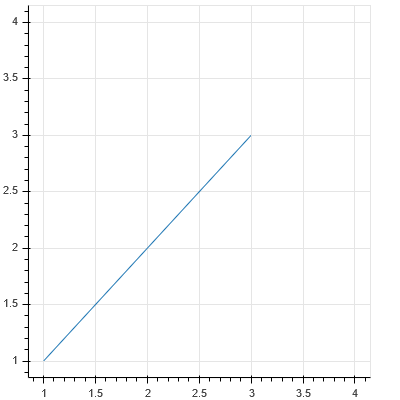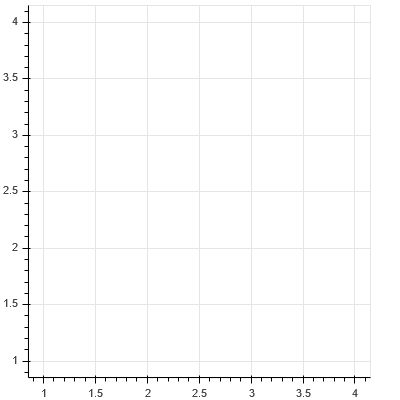# CDSView producing empty plots with GroupFilter or some sequences of indices in IndexFilter

I’m working in Anaconda 5/Python 3.6 on a Windows 10 system with Bokeh 0.12.7, looking at things in Firefox 55.0.3, 64-bit everything. I have a bunch of data I’d like to use subsets of to make a variety of plots, and from the docs it looks like CDSview filters would be the most direct way to go, but I’m having minimal success with them.

Example code for a thing that produces the expected line from points (1,1) to (3,3):

from bokeh import plotting,models

data = {‘x_vals’:[1,2,3,4],
‘y_vals’:[1,2,3,4],
‘group’:[‘a’,‘b’,‘a’,‘c’]}

data_source = models.ColumnDataSource(data=data)
current_view = models.CDSView(source=data_source, filters=[models.IndexFilter([2,0])])
plotting.output_file(“test.html”)

plot = plotting.figure(plot_width=400, plot_height=400)
plot.line(x=‘x_vals’,y=‘y_vals’,source=data_source,view=current_view)
plotting.show(plot)

``If I change the order of the list I’m feeding to IndexFilter it instead produces a plot with no line:

from bokeh import plotting,models

data = {‘x_vals’:[1,2,3,4],
‘y_vals’:[1,2,3,4],
‘group’:[‘a’,‘b’,‘a’,‘c’]}

data_source = models.ColumnDataSource(data=data)
current_view = models.CDSView(source=data_source, filters=[models.IndexFilter([0,2])])
plotting.output_file(“test.html”)

plot = plotting.figure(plot_width=400, plot_height=400)
plot.line(x=‘x_vals’,y=‘y_vals’,source=data_source,view=current_view)
plotting.show(plot)

``Attempting to use GroupFilter to select points (1,1) and (3,3) also produces an empty plot:

from bokeh import plotting,models

data = {‘x_vals’:[1,2,3,4],
‘y_vals’:[1,2,3,4],
‘group’:[‘a’,‘b’,‘a’,‘c’]}

data_source = models.ColumnDataSource(data=data)
current_view = models.CDSView(source=data_source, filters=[models.GroupFilter(column_name=‘group’,group=‘a’)])
plotting.output_file(“test.html”)

plot = plotting.figure(plot_width=400, plot_height=400)
plot.line(x=‘x_vals’,y=‘y_vals’,source=data_source,view=current_view)
plotting.show(plot)

``

Am I missing something obvious here or is this a bug somewhere in the filtering code? I expect the same output in all three cases.

Hi,

CDSView is a new feature that also happens to intersect Bokeh low level machinery. It is definitely the case that some kinks are still being worked out. There are some current PRs (one still open, one just merged) that address some open issues. My suggestion is to wait for the next "dev" build (a few days at most) with both the PRs merged, and see if they happen to resolve your issue as well. If not, a GH bug report would be appropriate at that time.

Thanks,

Bryan

···

On Sep 29, 2017, at 11:02, Karl Kuntzelman <[email protected]> wrote:

I'm working in Anaconda 5/Python 3.6 on a Windows 10 system with Bokeh 0.12.7, looking at things in Firefox 55.0.3, 64-bit everything. I have a bunch of data I'd like to use subsets of to make a variety of plots, and from the docs it looks like CDSview filters would be the most direct way to go, but I'm having minimal success with them.

Example code for a thing that produces the expected line from points (1,1) to (3,3):

from bokeh import plotting,models

data = {'x_vals':[1,2,3,4],
'y_vals':[1,2,3,4],
'group':['a','b','a','c']}

data_source = models.ColumnDataSource(data=data)
current_view = models.CDSView(source=data_source, filters=[models.IndexFilter([2,0])])
plotting.output_file("test.html")

plot = plotting.figure(plot_width=400, plot_height=400)
plot.line(x='x_vals',y='y_vals',source=data_source,view=current_view)
plotting.show(plot)

<Auto Generated Inline Image 1.png>

If I change the order of the list I'm feeding to IndexFilter it instead produces a plot with no line:

from bokeh import plotting,models

data = {'x_vals':[1,2,3,4],
'y_vals':[1,2,3,4],
'group':['a','b','a','c']}

data_source = models.ColumnDataSource(data=data)
current_view = models.CDSView(source=data_source, filters=[models.IndexFilter([0,2])])
plotting.output_file("test.html")

plot = plotting.figure(plot_width=400, plot_height=400)
plot.line(x='x_vals',y='y_vals',source=data_source,view=current_view)
plotting.show(plot)

<Auto Generated Inline Image 2.png>

Attempting to use GroupFilter to select points (1,1) and (3,3) also produces an empty plot:

from bokeh import plotting,models

data = {'x_vals':[1,2,3,4],
'y_vals':[1,2,3,4],
'group':['a','b','a','c']}

data_source = models.ColumnDataSource(data=data)
current_view = models.CDSView(source=data_source, filters=[models.GroupFilter(column_name='group',group='a')])
plotting.output_file("test.html")

plot = plotting.figure(plot_width=400, plot_height=400)
plot.line(x='x_vals',y='y_vals',source=data_source,view=current_view)
plotting.show(plot)

Am I missing something obvious here or is this a bug somewhere in the filtering code? I expect the same output in all three cases.
--
You received this message because you are subscribed to the Google Groups "Bokeh Discussion - Public" group.
To unsubscribe from this group and stop receiving emails from it, send an email to [email protected].
To post to this group, send email to [email protected].
To view this discussion on the web visit https://groups.google.com/a/continuum.io/d/msgid/bokeh/b1397001-40cd-4e07-a86f-6082659e2747%40continuum.io.